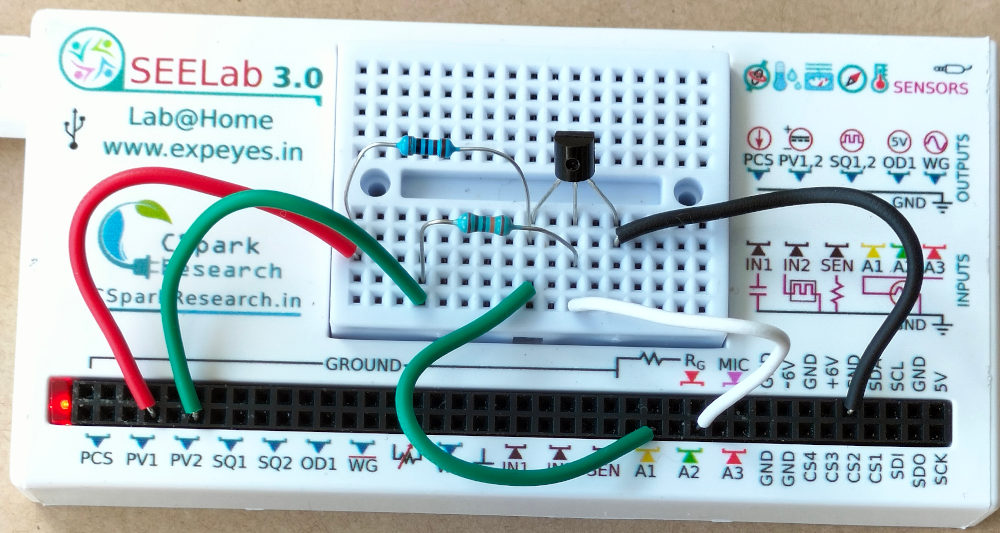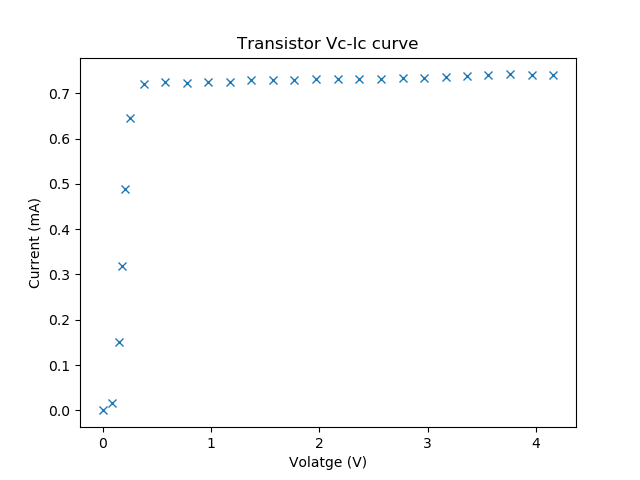# ExpEYES

## Output characteristics of an NPN transistor

The base current is set by the voltage from PV2, through a 100k resistor. The base voltage is measured to calculate the base current from Ib = (PV2-A2)/100K. The collector voltage is monitored by A1. The collector is connected to PV1, through a 1k resistor. For a selected base current, the voltage at PV1 is incremented in steps and at each step the collector voltage is measured. Corresponding collector current is calculated from i = (PV1-A1)/R. The transistor used is 2N2222, having a current gain of around 200. The schematic and photograph of the experimental setup and the results are shown below.### Python Program to generate the characteristics

Steps to perform this experiment manually are:

• Set the base current, by applying a volatge via the 100k resistor connected to the base.
• Set the voltage applied to the Collecter Vset, via the 1k resistor.
• Read the collector volatge Vc. Calculate Ic = (Vset-Vc)/R
• Increment Vset and repeat it until Vset is 5 volts (limit of our DC supply)
• Plot Vc against Ic
• Repeat the whole process for another base current.

The Python Program listed below implements the same steps.

import matplotlib.pyplot as plt

import eyes17.eyes
p = eyes17.eyes.open()

R = 1  # in kOhms, gives current in mA

for vb in [1,1.5,2]:
p.set_pv2(vb)		# Set the base current
x = []
y = []
v = 0.1
while v < 5:
p.set_pv1(v)
vd = p.get_voltage('A1')
i = (v-vd)/R
x.append(vd)
y.append(i)
print (vd, i)
v += 0.1
plt.plot(x,y)

plt.show()


Output of the program.